### Home > GC > Chapter 7 > Lesson 7.3.1 > Problem7-104

7-104.
1. For each pair of triangles below, determine if the triangles are congruent. If the triangles are congruent, state the congruence property that justifies your conclusion. If you cannot conclude that the triangles are congruent, explain why not. Homework Help ✎

1. ΔCAB ≅ Δ ____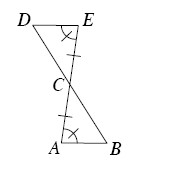2. ΔCBD ≅ Δ ____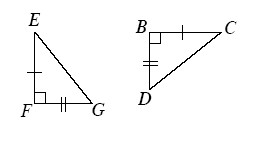3. ΔLJI ≅ Δ ____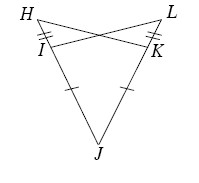4. ΔPRQ ≅ Δ ____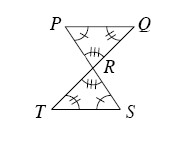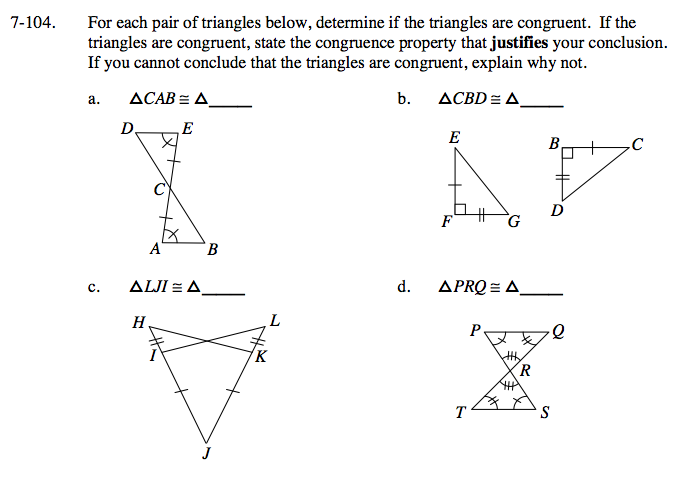ΔCED; vertical angles are equal, ASA≅

ΔEFG by SAS≅